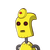# Which term of the A.P. 3, 14, 25, 36, … will be 99 more than its 25th term?​

Which term of the A.P. 3, 14, 25, 36, … will be 99 more than its

25th term?​

### 1 thought on “Which term of the A.P. 3, 14, 25, 36, … will be 99 more than its <br /><br />25th term?​”

1.## $$\huge \mathfrak \red{Æñßwēr}$$

Step-by-step explanation:

Let a be the first term and d be the common difference.

Now, first term,a= 3

$$common \: difference, \: d = a2 – a = 14 – 3= 11$$

## $$⇒n = \frac{374}{11} = 34$$

$$hence \: 34 ^{th} \: term \: of \: AP \: is \: 99 \: more \: than \: {25}^{th} \: term$$